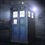# Integration problems, help!

Prove that,$\int_{0}^{\infty}\dfrac{1}{x^3}\sin^3\left(x-\dfrac{1}{x} \right)^5\, dx=0$ and$\int_{-1}^{0}\dfrac{x^2+2x}{\ln{(x+1)}} dx=\ln{3}$.I have no idea how to solve these problems!6 years ago

This discussion board is a place to discuss our Daily Challenges and the math and science related to those challenges. Explanations are more than just a solution — they should explain the steps and thinking strategies that you used to obtain the solution. Comments should further the discussion of math and science.

When posting on Brilliant:

• Use the emojis to react to an explanation, whether you're congratulating a job well done , or just really confused .
• Ask specific questions about the challenge or the steps in somebody's explanation. Well-posed questions can add a lot to the discussion, but posting "I don't understand!" doesn't help anyone.
• Try to contribute something new to the discussion, whether it is an extension, generalization or other idea related to the challenge.

MarkdownAppears as
*italics* or _italics_ italics
**bold** or __bold__ bold
- bulleted- list
• bulleted
• list
1. numbered2. list
1. numbered
2. list
Note: you must add a full line of space before and after lists for them to show up correctly
paragraph 1paragraph 2

paragraph 1

paragraph 2

[example link](https://brilliant.org)example link
> This is a quote
This is a quote
    # I indented these lines
# 4 spaces, and now they show
# up as a code block.

print "hello world"
# I indented these lines
# 4 spaces, and now they show
# up as a code block.

print "hello world"
MathAppears as
Remember to wrap math in $$ ... $$ or $ ... $ to ensure proper formatting.
2 \times 3 $2 \times 3$
2^{34} $2^{34}$
a_{i-1} $a_{i-1}$
\frac{2}{3} $\frac{2}{3}$
\sqrt{2} $\sqrt{2}$
\sum_{i=1}^3 $\sum_{i=1}^3$
\sin \theta $\sin \theta$
\boxed{123} $\boxed{123}$

Sort by:

- 6 years ago

Hello Adarsh. I'm really sorry but I won't be able to help now; I'm truly exhausted after coaching class since 10 in the morning - and the same schedule is in place for tomorrow. I'll answer as soon as I can for sure.

- 6 years ago

No problem bhaiya!I understand!

- 6 years ago

Hint about second integral, take x+1=t, and do it with the help of differentiation under integral.

- 6 years ago

Actually i tried that and I got,$\int_{0}^{1}\dfrac{t^2-1}{\ln{t}}dt$,how to proceed now?

- 6 years ago

@Adarsh Kumar Seems you've got some excellent answers. I would have done the second the same way as Rajdeep.

- 6 years ago

Sir the 1st one ? @Samarpit Swain solution is wrong.

- 6 years ago

Come on Rajdeep! I'm not Sir!I'll have a go at it in half an hour.

- 6 years ago

Yes sir U are "sir" for me.

- 6 years ago

By the way really nice solution!

- 6 years ago

Thank You sir

- 6 years ago

@Adarsh Kumar @Rajdeep Dhingra The first Integral is really hard. Also, it is not equal to $0.$ The Integral is equivalent to $-\frac15 \int_0^\infty \sin^3(u) u^{-3/5} du \approx {-0.1535}$ Anyway, to be able to compute the original Integral, you need to know some conditions for which an Integral becomes Riemann Improper Integrable. I can try to proceed if you want, but this is really miles and miles above our level.

- 6 years ago